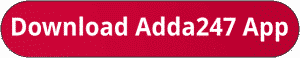Latest Banking jobs   »   sbi quant

# Quantitative Aptitude Quiz For SBI Clerk Prelims 2023 -09th November

Q1. The breadth and length of a rectangle are in the ratio of 2:7. If the perimeter of rectangle is 198 cm, then find the area of rectangle? (in cm²)
(a)1576
(b)2214
(c)1876
(d)2108
(e)1694

Q2. The ratio of speed of two trains which are running in the same direction is 3 : 5. The train having higher speed crosses the second train in 45 seconds and a pole in 6 seconds respectively. Find the ratio of their lengths?
(a) 2 : 1
(b) 2 : 3
(c) 3 : 5
(d) 4 : 5
(e) 6 : 7

Q3. In a society 16 ⅔% people were interested in music only, 33⅓% were interested in Yoga only, 25% were interested in cycling only and rest were interested in other activities. If no. of people who are interested in other activities is 450, then find the number of people who are interested in music only?
(a) 400
(b) 300
(c) 250
(d) 350
(e) 450

Q4. A man purchases three types of items in ratio 5:3:6 and their cost price are Rs. 100, Rs. 400 and Rs. 200 respectively. If he sold first, second and third type of article at 20%, 15% and 25% profit respectively, then find his overall profit percentage?
(a) 40%
(b) 32%
(c) 24%
(d) 15%
(e) 20%

Q5. Find the ratio of volume of cylinder ‘A’ to volume of cylinder ‘B’, if ratio of radius of cylinders ‘A’ to cylinder ‘B’ is 2: 3 while the ratio of height of cylinder ‘A’ to cylinder ‘B’ is 5 : 4?
(a) 1 : 1
(b) 5 : 9
(c) 9 : 4
(d) 3 : 4
(e) 4 : 5

Q6. If the ratio of marked price to cost price of an item is 8:5 and the discount offered is 20% on the item, then find the profit/loss percentage.
(a)28%
(b)15%
(c)20%
(d)36%
(e)32%

Q7. If the maximum marks in a test is 600 and the passing percentage is 33⅓% and a student scores 155 marks, then find the marks needed by student to pass the test?
(a)25
(b)45
(c)30
(d)35
(e)15

Q8. A fraction becomes 5/8 when 25% of numerator is added to its numerator and 40% of its denominator is subtracted from its denominator. Find the fraction?
(a) 3/10
(b) 6/25
(c) 3/35
(d) 2/35
(e) 35/33

Q9. Raheem spends 24% of his monthly income on daily spendings, 36% on house rent and children fee together and rest amount he saves for future needs. If his total savings be Rs. 12,800, then find his total monthly income?
(a) Rs. 45,000
(b) Rs. 40,000
(c) Rs. 48,000
(d) Rs. 32,000
(e) Rs. 50,000

Q10. The ratio between perimeter of an equilateral triangle and a square is 1 : 4. The area of square is 200% of area of a rectangle having sides 16 m and 8 m. Find the perimeter of triangle?
(a) 22 m
(b) 20 m
(c) 16 m
(d) 28 m
(e) 32 m

Solutions.                                                           .                                           .

## FAQs

### What are sections included in the SBI Clerk Prelims Exam?

Reasoning Ability, Numerical Ability and English sections are included in the SBI Clerk Prelims Exam

#### Congratulations!Union Budget 2023-24: Free PDF